# Fractions, types of fractions

In addition to the natural numbers and zero, there are other numbers - fractional numbers. When one object is divided ( Apple, cake, sheet of paper ) or the unit of measurement ( meter, kilogram, degree ) divided into equal parts, there are fractional numbers.

Half, quarter, one third, one hundredth are examples of rational numbers.

Definition: a fraction (or simple fraction)is a number represented in the form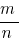where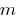is an integer, and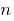natural.

Entry form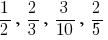fractions, or in short just a fraction.

The numerator of the fraction is the number written above the line shot.

Denominator — the number written below the line shot.

The denominator shows how many equal parts the whole is divided. And the numerator shows how many parts have taken.

## The fundamental property of fractions

If the numerator and denominator of a fraction be multiplied or divided by the same amount that is not equal to zero, then the resulting fraction will be equal to the initial, although the fractions are different.

For example,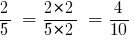And, conversely,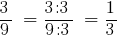## Proper and improper fractions

Can a numerator equal to the denominator? Yes, you can! For example, pramodini divided into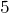equal parts, and painted everything. So, it turned out the shaded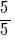rectangle, is equal to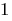, or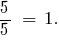Definition: If in fractions the numerator is less than denominator the fraction is called proper fraction.

Definition: If the normal of a fraction the numerator more than the denominator then the fraction is called improper fraction.

Fractions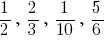— proper fractions.

Fractions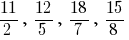— improper fractions.

### Compare fractions

Of two fractions with equal denominators more about who has the numerator bigger.

For Example,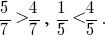As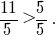So

All proper fractions are less than unity, and the wrong is greater than or equal to one. Each nepravilni fraction more correct!

## A mixed fraction. A mixed number

Improper fractions are mixed numbers. Any improper fraction can be presented in the form of natural numbers or the sum of a natural number and a proper fraction.

The number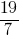can be written as sum of two fractions, for example:.

Since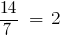then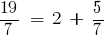The amount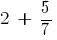is usually written more brieflyThe number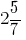is called a mixed number. Thus the natural number 2 is called the integer part of the fraction, and proper fraction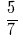a fractional part of the fraction.

Definition: a Mixed number is a number which is recorded in the form of a whole number and proper fraction is understood as the sum of this number and fractions.

### How to turn an improper fraction into a mixed number

To improper fraction convert to a mixed number, the numerator is not evenly divided by the denominator, the numerator must be divided into Znamenny. Received incomplete share will be the integer part of the mixed number and the remainder is the numerator of its fractional part.

Example: Translate the improper fractionto a mixed number.

Solutions:

Divide the numerator by the denominator. Happened: a 29 - whole number portion and the remainder is 3. So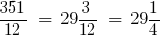### How to turn a mixed number into an improper fraction

To a mixed number to turn into an improper fraction, you need the integer part multiply the fractional part Znamenny and received doboduto the numerator of the fractional part. The resulting sum is the numerator improper fractions, and its denominator is the denominator of the fractional part of the mixed number.

Example: Convert the mixed number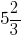to an improper fraction.

Solutions:

Turn: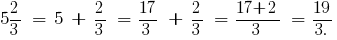But do not worry, in the near future cubens.com retrobyte calculators that will help You decide.

Tags:
Chapter:
Versions in other languages:
Share with friends: